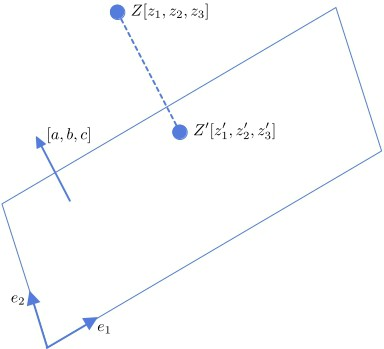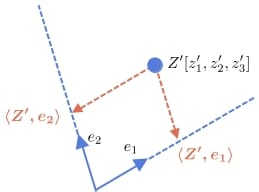## 1. Introduction

In this tutorial, we’ll explain how to project a three-dimensional point onto a (two-dimensional) plane with its coordinate system.

## 2. Setup

Let’s say we have a point with coordinatesand a plane whose equation is:

(1)whereis any point in the plane, andare its parameters. Geometrically,is the vector normal to the plane. It doesn’t have to be unitary but needs to be non-zero. Parameteris the-coordinate of the plane’s intersection with the-axis. Visually:The plane has a two-dimensional coordinate system of its own, defined by two base unit vectorsand.

So, our goal is to find the coordinates of the‘s projectiononto the plane in the coordinate system defined byand.

## 3. How to Project a Point

Let’s start by projectingonto the plane and finding the coordinates in the original 3D system. Since the projectionbelongs to the plane, its coordinates fit Equation (1):

(2)Further, the line connectingandis perpendicular to the plane, so it’s parallel to the plane’s normal vector. The line’s vector is. So, the following should hold:

(3)for some real number.

By solving (3) for,, and, and plugging the obtained expressions into Equation (2), we get:If the normal vector is also a unit vector (i.e., its length is 1), the denominator is also one since it denotes the vector’s squared length.

By substitutingin Equations (2), we can easily calculate the coordinates:### 3.1. Example

Letand let the plane’s equation be:For, we get:So, the projectionis.

### 3.2. An Alternative Parameterization of the Plane

The plane can be specified by the normal vectorand a pointin it. For an arbitrary pointin the plane, it must hold that the vectoris perpendicular to:

Therefore:This is the same as Equation (??) if we set. So, after computing, we can proceed as in the sections above.

## 4. Finding New Coordinates

We get the new coordinates ofby projecting it onto the unit vectorsand:To do that, it’s sufficient to find the dot productsand.

Now, let’s suppose thatand. If we treat all the vectors (including points) as column vectors, we can get the new coordinates ofby matrix multiplication:

(4)### 4.1. Example

Let’s say thatis,, and:

The coordinates ofin the new coordinate system are.

## 5. Conclusion

In this article, we showed how to project a point onto a plane and find its coordinates in the plane’s coordinate system.